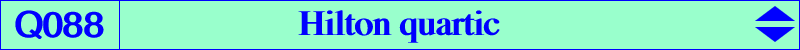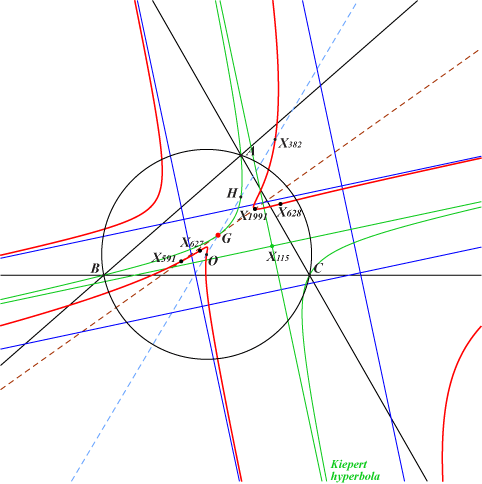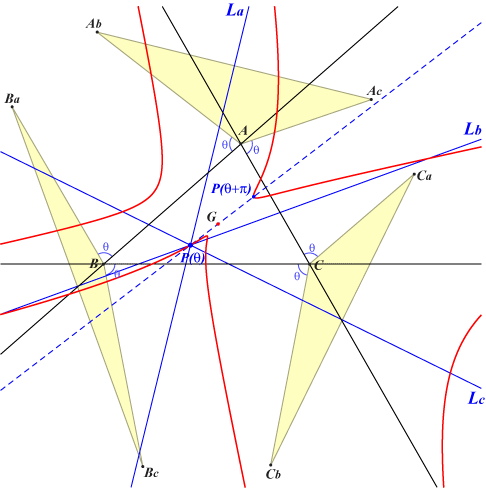too complicated to be written here. Click on the link to download a text file.X(2), X(3), X(382), X(627), X(628), X(1991), X(3095), X(3413), X(3414) X(22113), X(22114) anticomplements of X(627), X(628) resp. imaginary foci of the Brocard ellipse i.e. common points of the Brocard axis and the Kiepert hyperbola(contributed by Richard Hilton) Let each side of a triangle be rotated about each of its end-vertices, by equal angles θ, externally if θ is positive. Next let the outer ends be joined for each pair of segments that share a common vertex. Then the perpendicular bisectors La, Lb, Lc of these joins are concurrent, at P(θ), as in the diagram. Adding π to θ is equivalent to reflecting each of the joining segments in the corresponding vertex of the reference triangle, so that the mid-point M(θ) of P(θ) and P(θ+π) is the point of concurrency of the lines through the vertices that are perpendicular to the joining segments. This point M(θ) lies on the Kiepert hyperbola. Also P(θ) and P(θ+π) are always collinear with the centroid G. The locus of P(θ) as θ varies is the quartic Q088 with two pairs of parallel asymptotes, each pair having an asymptote of the Kiepert hyperbola as mid-line. The locus is shown above, together with the Kiepert hyperbola and asymptotes. Note that G is an acnodal point on the curve.Additional properties • The line M(θ)M(-θ) contains K and bisects the parallel lines P(θ)P(-θ), P(π+θ)P(π-θ). • The line P(θ)P(-θ) envelopes a hyperbola (H) with center K passing through X(511), X(524), the midpoints of X(3)X(141), X(69)X(182), X(193)X(576). This hyperbola is quadritangent to Q088. • The lines P(θ)P(π-θ) and P(-θ)P(π+θ) meet at S(θ). The locus of S(θ) is a hyperbola (H') passing through X(2), X(39), X(99), X(141), X(550), X(2896), X(3098), X(3413), X(3414). • The midpoints of P(θ)P(-θ) and P(π+θ)P(π-θ) lie on the line through G and S(θ).